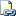# CONCAT (Transact-SQL)

Returns a string that is the result of concatenating two or more string values.Transact-SQL Syntax Conventions

## Syntax

``````CONCAT ( string_value1, string_value2 [, string_valueN ] )
``````

## Arguments

• string_value
A string value to concatenate to the other values.

## Return Types

String, the length and type of which depend on the input.

## Remarks

CONCAT takes a variable number of string arguments and concatenates them into a single string. It requires a minimum of two input values; otherwise, an error is raised. All arguments are implicitly converted to string types and then concatenated. Null values are implicitly converted to an empty string. If all the arguments are null, an empty string of type varchar(1) is returned. The implicit conversion to strings follows the existing rules for data type conversions. For more information about data type conversions, see CAST and CONVERT (Transact-SQL).

The return type depends on the type of the arguments. The following table illustrates the mapping.

Input type

Output type and length

If any argument is a SQL-CLR system type, a SQL-CLR UDT, or nvarchar(max)

nvarchar(max)

Otherwise, if any argument is varbinary(max) or varchar(max)

varchar(max) unless one of the parameters is an nvarchar of any length. If so, then the result is nvarchar(max).

Otherwise, if any argument is nvarchar(<= 4000)

nvarchar(<= 4000)

Otherwise, in all other cases

varchar(<= 8000)unless one of the parameters is an nvarchar of any length. If so, then the result is nvarchar(max).

When the arguments are <= 4000 for nvarchar, or <= 8000 for varchar, implicit conversions can affect the length of the result. Other data types have different lengths when they are implicitly converted to strings. For example, an int (14) has a string length of 12, while a float has a length of 32. Thus the result of concatenating two integers has a length of no less than 24.

If none of the input arguments is of a supported large object (LOB) type, then the return type is truncated to 8000 in length, regardless of the return type. This truncation preserves space and supports efficiency in plan generation.

This function is capable of being remoted to SQL Server 2012 servers and above. It will not be remoted to servers that have a version below SQL Server 2012.

## Examples

### A. Using CONCAT

``````SELECT CONCAT ( 'Happy ', 'Birthday ', 11, '/', '25' ) AS Result;
``````

Here is the result set.

``````Result
-------------------------
Happy Birthday 11/25

(1 row(s) affected)
``````

### B. Using CONCAT with NULL values

``````CREATE TABLE #temp (
emp_name nvarchar(200) NOT NULL,
emp_middlename nvarchar(200) NULL,
emp_lastname nvarchar(200) NOT NULL
);
INSERT INTO #temp VALUES( 'Name', NULL, 'Lastname' );
SELECT CONCAT( emp_name, emp_middlename, emp_lastname ) AS Result
FROM #temp;
``````

Here is the result set.

``````Result
------------------
NameLastname

(1 row(s) affected)
``````